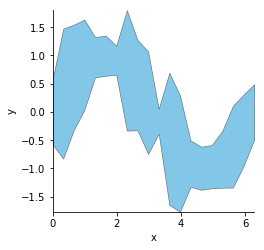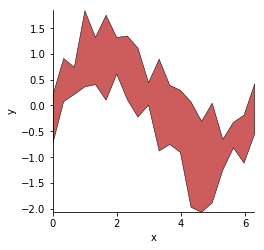Title
Dependencies
Matplotlib
Backends
Bokeh
Matplotlib
Plotly
In :
import numpy as np
import holoviews as hv
hv.extension('matplotlib')Spread elements have the same data format as the ErrorBars element, namely x- and y-values with associated symmetric or asymmetric errors, but are interpreted as samples from a continuous distribution (just as Curve is the continuous version of Scatter). These are often paired with an overlaid Curve to show an average trend along with a corresponding spread of values; see the Tabular Datasets user guide for examples.

Note that as the Spread element is used to add information to a plot (typically a Curve) the default alpha value is less that one, making it partially transparent.

##### Symmetric¶

Given two value dimensions corresponding to the position on the y-axis and the error, Spread will visualize itself assuming symmetric errors:

In :
np.random.seed(42)
xs = np.linspace(0, np.pi*2, 20)
err = 0.2+np.random.rand(len(xs))

Out:##### Asymmetric¶

Given three value dimensions corresponding to the position on the y-axis, the negative error and the positive error, Spread can be used to visualize assymmetric errors:

In :
xs = np.linspace(0, np.pi*2, 20)For full documentation and the available style and plot options, use hv.help(hv.Spread).